# Cubic Graph

Here we will learn about cubic graphs, including recognising and sketching cubic graphs. We will also look at plotting and interpreting cubic graphs.

There are also cubic graphs worksheets based on Edexcel, AQA and OCR exam questions, along with further guidance on where to go next if you’re still stuck.

## What is a cubic graph?

A cubic graph is a graphical representation of a cubic function.

A cubic is a polynomial which has an x3 term as the highest power of x.

Some cubic graphs have two turning points –  a minimum point and a maximum point. A cubic graph with two turning points can touch or cross the x axis between one and three times.

E.g.

In the graph below the coefficient of the x^3 term is positive so the graph increases

y=x^{3}-2x^{2}-x+2

Also, the y -intercept of the curve is +2 and the equation ends with +2 for when x=0.

### What is a cubic graph?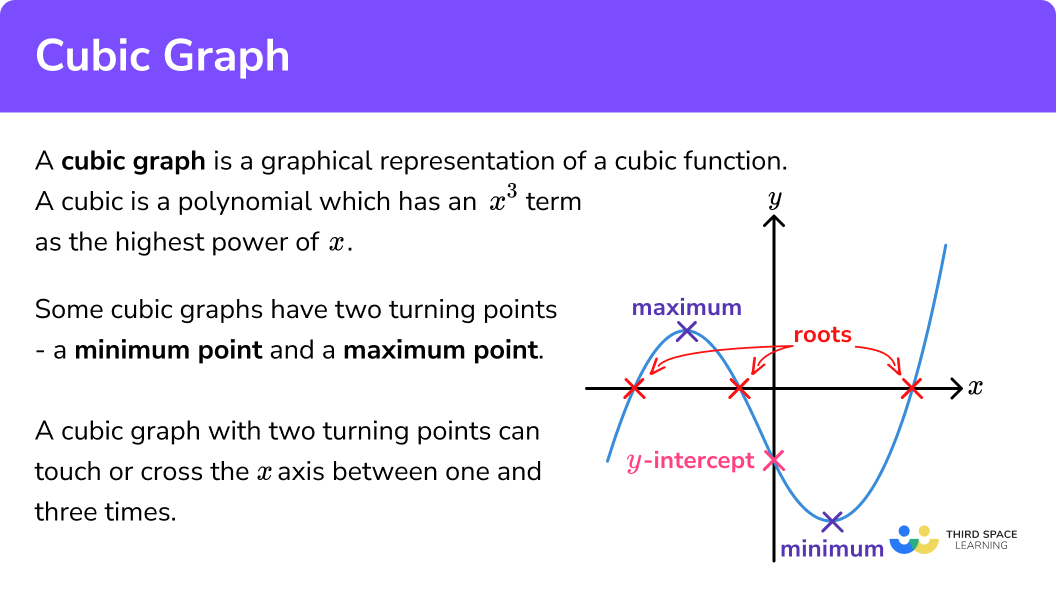E.g.

In the graph below the coefficient of the x^3 term is negative so the graph decreases

y=-x^{3}+2x^{2}+x-2

Also, the y -intercept of the curve is -2 and the equation ends with -2 for when x=0.

Some cubic graphs have one turning point.

E.g.

This is graph of y=x^3

### What is a cubic graph?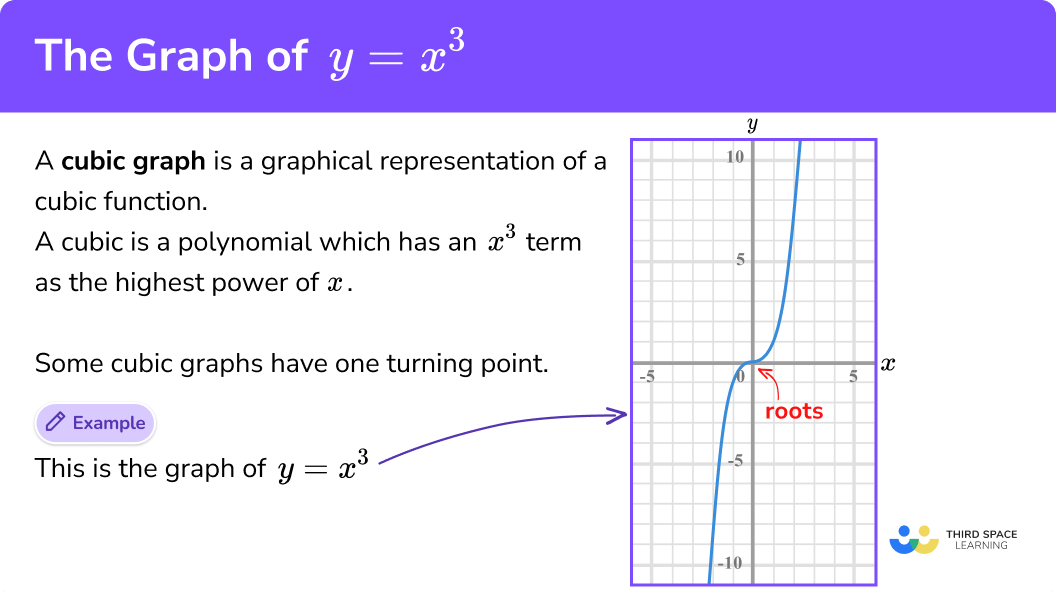## How to identify a cubic graph

In order to recognise a cubic graph:

1. Identify linear or quadratic or any other functions.
2. Identify the cubic function checking if the x3 term is positive or negative.

Cubic graph is part of our series of lessons to support revision on types of graphs. You may find it helpful to start with the main types of graphs lesson for a summary of what to expect, or use the step by step guides below for further detail on individual topics. Other lessons in this series include:

## Identifying a cubic graph examples

### Example 1: recognising cubic graphs

Identify the correct graph for the equation:

$y=x^3+2x^2+7x+4$

1. Identify linear or quadratic or any other functions.

Graph A is a straight line – it is a linear function.

Graph B is a parabola – it is a quadratic function.

2Identify the cubic function checking if the x3 term is positive or negative.

Graph C and Graph D could be cubic functions.

The given equation has a positive x3 term.

For Graph C the graph decreases, so Graph C cannot be the correct graph.

Graph D is increasing.

Graph D is the correct graph for the equation.

### Example 2: recognising cubic graphs

Identify the correct graph for the equation:

$y=-x^3+2x^2+4$

Graph B is a straight line – it is a linear function

Graph D is a parabola – it is a quadratic function

Graph A and Graph C could be cubic functions. They have two turning points.

The given equation has a negative x3 term so the graph will decrease.

Graph C is increasing, so Graph C cannot be the correct graph.

Graph A is decreasing.

Graph A is the correct graph for the equation.

## How to plot a cubic graph

In order to plot a cubic graph:

1. Complete the table of values.
2. Plot the coordinates.
3. Draw a smooth curve through the points.

### Explain how to plot a cubic graph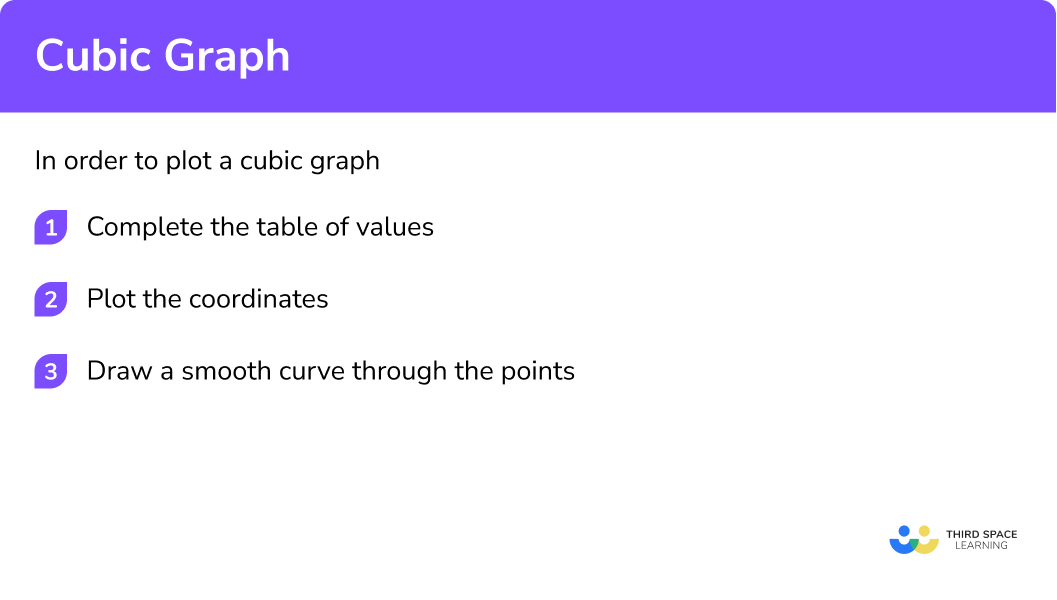## Plotting a cubic graph examples

### Example 3: draw the curve of the equation for −2 ≤ x ≤ 2

$y=x^{3}-3x-1$

Draw an x-axis and a y-axis. It is best practice to use a pencil and plot the coordinates using small crosses.

The coordinates would be (−2,−3), (−1,1) and so on.

Use a pencil and turn the paper around if it makes it easier for you.

Note: the y-intercept of the curve is −1 and the equation ends with −1 for when x = 0.

### Example 4: draw the curve of the equation for −3 ≤ x ≤ 3

$y=x^3-6x+1$

Draw an x-axis and a y-axis.
It is best practice to use a pencil and plot the coordinates using small crosses.

Use a pencil and turn the paper around if it makes it easier for you.

Note: the y-intercept of the curve is +1 and the equation ends with +1 for when
x = 0.

## How to use a cubic graph

In order to use a cubic graph to solve an equation:

1. Find the given value on the y-axis.
2. Draw a straight horizontal line across the curve.
3. Draw a straight vertical line from the curve to the x-axis.
4. Read off the value on the x-axis.

## Using a cubic graph examples

### Example 5: use a cubic graph to solve the equation

Use the graph of y = x^3 - 5x + 1 to find an approximate solution of the following equation:

$x^3-5x+1=6$

The value of 6 is in place of the y
We need to look on the y-axis for the value of 6.

Make sure you use a ruler and be as accurate as you can.

Continue to use a ruler and try to be as accurate as you can.

Be careful with the scale on x-axis.  The solution to the equation is only approximate, but try to be as accurate as you can.

x = 2.6

You could substitute the x value back into the original equation to check.

### Example 6: use a cubic graph to solve the equation

Use the graph of y = x^3 - 7x - 3 to find approximate solutions of the following equation:

$x^3-7x-3=2$

The value of 2 is in place of the y.  We need to look on the y-axis for the value of 2.

Make sure you use a ruler and be as accurate as you can. The horizontal line intersects the curve in 3 places.

Continue to use a ruler and try to be as accurate as you can.  There are 3 vertical lines required here.

Be careful with the scale on x-axis.  There are 3 different x values to read off. The solutions to the equation are only approximate, but try to be as accurate as you can.

x= -2.2, -0.8 and 2.9

### Common misconceptions

• Scale on the x and y axis

Cubic graphs often have different scales on the x-axis and the y-axis.  So be careful when you are plotting coordinates.

• The curve must be smooth

Cubic graphs need to be drawn with a smooth curve. Avoid straight line segments.

Incorrect:

Correct:

• Cubic graphs have at the most only 2 turning points

Make sure that your cubic graph has only one minimum point and one maximum point.  Check that you have the correct values and that you have plotted them accurately.

• You can use the y-intercept to help distinguish between cubic curves

The y-intercept is where the curve crosses the y-axis.  It is also easy to spot on an equation.  The following equation ends with a +4 and this will be the y-intercept of the graph.

$y=x^3+x^2+4$

### Practice cubic graph questions

1. Identify the correct graph for the below equation:

y=x^3-3x+2

Graph A:Graph  B:Graph C:Graph D:Graph AGraph BGraph CGraph DGraph A is a growth curve so is an exponential function

Graph B has 2 turning points so could be the graph of a cubic function.

Graph C is a parabola so is a quadratic function.

Graph D is a hyperbola so is a reciprocal function.

Graph B is the only curve which could be a cubic function.

Also the y -intercept is positive on the curve and the equation.

2. Identify the correct graph for the equation:

y=-x^3+2x-3

Graph A:Graph B:Graph C:Graph D:Graph AGraph BGraph CGraph DAll graphs have two turning points so all the graphs could be graphs of cubic functions.

Graph B and Graph C show that as x increases so does y so they have a positive x^3 term. So the correct graph is not one of these.

Graph A and Graph D show that as x increases so does y but negatively so they have a negative x^3 term.

But the y -intercept on Graph A is negative, and the given equation ends with -3 so this is the correct graph.3. Identify the correct graph for the equation:

y=x^{3}-2x+3
Graph A:Graph B&nbso;
Graph C:Graph D:Graph AGraph BGraph CGraph DA correct table of values would be:

These values give coordinates such as (-2,-1), (-1,4) and so on.

The coordinates are plotted on the grid.

A smooth curve should be drawn through the points.

4. Identify the correct graph for the equation:

y=x^3-4x+5
Graph A:Graph B:Graph C:Graph D :Graph AGraph BGraph CGraph DA correct table of values would be:

These values give coordinates such as (-3,-10), (-2,5) and so on.

The coordinates are plotted on the grid.

A smooth curve should be drawn through the points.

5. Use the graph of y=6+3x-x^3 to solve:

6+3x-x^3=22.22.52.91.96. Use the graph of y=x^3-3x+7 to solve:

x^3-3x+7=4-2.12.1-1.91.9### Cubic graph GCSE questions

1.  Match the correct equation to the graph:(1 mark)

y=x^3-5x+2

(1)

2.

(a) Complete the table of values for y=x^{3}-8x+3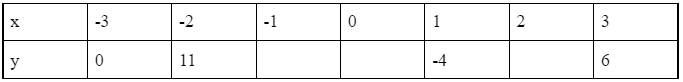(b) On the grid, draw the graph of y=x^{3}-8x+3 for

-3\leq{x}\leq3(4 marks)

(a)

Correct values are: 10, 3 and -5.

For 1 correct y -values.

(1)

For all correct y -values.

(1)

(b)For plotting the points correctly.

(1)

For the smooth curve.

(1)

3.

(a) Complete the table of values for y=x^{3}-6x+2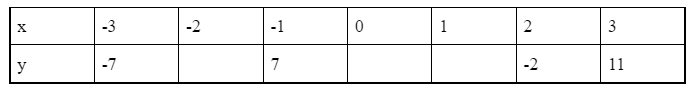(b) On the grid, draw the graph of y=x^{3}-6x+2 for

-3\leq{x}\leq3(c) Use the graph to solve the equation:

x^3-6x+2=8

(5 marks)

(a)

Correct values are: 6, 2 and -3.

For 1 correct y -value.

(1)

For all correct y -values.

(1)

(b)For plotting the points correctly.

(1)

For the smooth curve.

(1)

(c)

For answer between 2.7 and 2.9.

(1)## Learning checklist

You have now learned how to:

• Recognise graphs of cubic functions
• Draw graphs of cubic functions
• Interpret graphs of cubic functions

## Still stuck?

Prepare your KS4 students for maths GCSEs success with Third Space Learning. Weekly online one to one GCSE maths revision lessons delivered by expert maths tutors.

Find out more about our GCSE maths tuition programme.